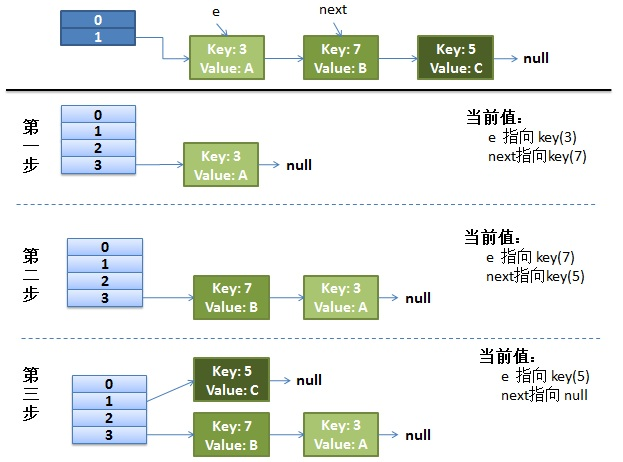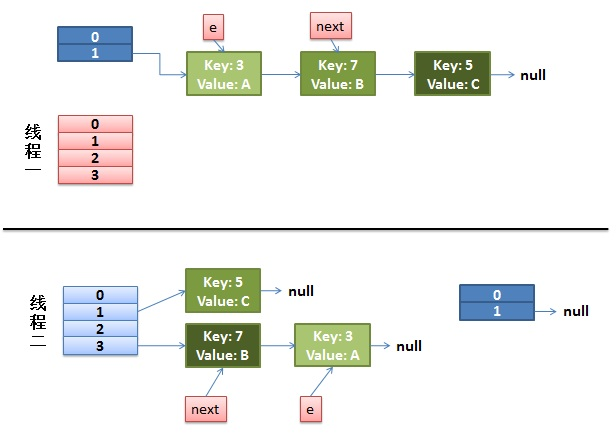# HashMap 基本实现（JDK 8 之前）

HashMap 通常会用一个指针数组（假设为 table[]）来做分散所有的 key，当一个 key 被加入时，会通过 Hash 算法通过 key 算出这个数组的下标 i，然后就把这个 <key, value> 插到 table[i] 中，如果有两个不同的 key 被算在了同一个 i，那么就叫冲突，又叫碰撞，这样会在 table[i] 上形成一个链表# HashMap 的 rehash 源代码

Put 一个 Key, Value 对到 Hash 表中：

``````public V put(K key, V value) {
......
// 计算 Hash 值
int hash = hash(key.hashCode());
int i = indexFor(hash, table.length);
// 如果该 key 已被插入，则替换掉旧的 value（链接操作）
for (Entry<K,V> e = table[i]; e != null; e = e.next) {
Object k;
if (e.hash == hash && ((k = e.key) == key || key.equals(k))) {
V oldValue = e.value;
e.value = value;
e.recordAccess(this);
return oldValue;
}
}
modCount++;
// 该 key 不存在，需要增加一个结点
return null;
}
``````

``````void addEntry(int hash, K key, V value, int bucketIndex) {
Entry<K,V> e = table[bucketIndex];
table[bucketIndex] = new Entry<K,V>(hash, key, value, e);
//查看当前的 size 是否超过了设定的阈值 threshold，如果超过，需要 resize
if (size++ >= threshold)
resize(2 * table.length);
}
``````

``````void resize(int newCapacity) {
Entry[] oldTable = table;
int oldCapacity = oldTable.length;
......
// 创建一个新的 Hash Table
Entry[] newTable = new Entry[newCapacity];
// 将 Old Hash Table 上的数据迁移到 New Hash Table 上
transfer(newTable);
table = newTable;
}
``````

``````void transfer(Entry[] newTable) {
Entry[] src = table;
int newCapacity = newTable.length;
//下面这段代码的意思是：
//  从OldTable里摘一个元素出来，然后放到NewTable中
for (int j = 0; j < src.length; j++) {
Entry<K,V> e = src[j];
if (e != null) {
src[j] = null;
do {
Entry<K,V> next = e.next;
int i = indexFor(e.hash, newCapacity);
e.next = newTable[i];
newTable[i] = e;
e = next;
} while (e != null);
}
}
}
``````

# 正常的 ReHash 的过程

• 假设我们的 hash 算法就是简单的用 key mod 一下表的大小（也就是数组的长度）
• 最上面的是 old hash 表，其中的 Hash 表的 size = 2，所以 key = 3, 7, 5，在 mod 2 以后都冲突在 table 这里了
• 接下来的三个步骤是 Hash 表 resize 成 4，然后所有的 <key, value> 重新 rehash 的过程# 并发下的 Rehash

1）假设有两个线程

``````do {
Entry<K,V> next = e.next; //  假设线程一执行到这里就被调度挂起了
int i = indexFor(e.hash, newCapacity);
e.next = newTable[i];
newTable[i] = e;
e = next;
} while (e != null);
``````2）线程一被调度回来执行

• 先是执行 newTalbe[i] = e;
• 然后是 e = next，导致了 e 指向了 key(7)
• 而下一次循环的 next = e.next 导致了 next 指向了 key(3)3）线程一接着工作。把 key(7) 摘下来，放到 newTable[i] 的第一个，然后把 e 和 next 往下移4）环形链接出现
e.next = newTable[i] 导致 key(3).next 指向了 key(7)# JDK 8 的改进

JDK 8 中采用的是位桶 + 链表/红黑树的方式，当某个位桶的链表的长度超过 8 的时候，这个链表就将转换成红黑树

HashMap 不会因为多线程 put 导致死循环（JDK 8 用 head 和 tail 来保证链表的顺序和之前一样；JDK 7 rehash 会倒置链表元素），但是还会有数据丢失等弊端（并发本身的问题）。因此多线程情况下还是建议使用 ConcurrentHashMap

# 为什么线程不安全

HashMap 在并发时可能出现的问题主要是两方面：

• 如果多个线程同时使用 put 方法添加元素，而且假设正好存在两个 put 的 key 发生了碰撞（根据 hash 值计算的 bucket 一样），那么根据 HashMap 的实现，这两个 key 会添加到数组的同一个位置，这样最终就会发生其中一个线程 put 的数据被覆盖

• 如果多个线程同时检测到元素个数超过数组大小 * loadFactor，这样就会发生多个线程同时对 Node 数组进行扩容，都在重新计算元素位置以及复制数据，但是最终只有一个线程扩容后的数组会赋给 table，也就是说其他线程的都会丢失，并且各自线程 put 的数据也丢失

• 暂无文章